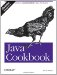# Recipe 5.6 Comparing Floating-Point Numbers

#### Problem

You want to compare two floating-point numbers for equality.

#### Solution

Based on what we've just discussed, you probably won't just go comparing two floats or doubles for equality. You might expect the floating-point wrapper classes, Float and Double, to override the equals( ) method, which they do. The equals( ) method returns true if the two values are the same bit for bit, that is, if and only if the numbers are the same or are both NaN. It returns false otherwise, including if the argument passed in is null, or if one object is +0.0 and the other is -0.0.

If this sounds weird, remember that the complexity comes partly from the nature of doing real number computations in the less-precise floating-point hardware, and partly from the details of the IEEE Standard 754, which specifies the floating-point functionality that Java tries to adhere to, so that underlying floating-point processor hardware can be used even when Java programs are being interpreted.

To actually compare floating-point numbers for equality, it is generally desirable to compare them within some tiny range of allowable differences; this range is often regarded as a tolerance or as epsilon . Example 5-1 shows an equals( ) method you can use to do this comparison, as well as comparisons on values of NaN. When run, it prints that the first two numbers are equal within epsilon:

`\$ java FloatCmp True within epsilon 1.0E-7  \$`

##### Example 5-1. FloatCmp.java
`}/**  * Floating-point comparisons.   */ public class FloatCmp {     final static double EPSILON = 0.0000001;     public static void main(String[] argv) {         double da = 3 * .3333333333;         double db = 0.99999992857;         // Compare two numbers that are expected to be close.         if (da == db) {             System.out.println("Java considers " + da + "==" + db);         // else compare with our own equals method         } else if (equals(da, db, 0.0000001)) {             System.out.println("True within epsilon " + EPSILON);         } else {             System.out.println(da + " != " + db);         }         // Show that comparing two NaNs is not a good idea:         double d1 = Double.NaN;         double d2 = Double.NaN;         if (d1 == d2)             System.err.println("Comparing two NaNs incorrectly returns true.");         if (!new Double(d1).equals(new Double(d2)))             System.err.println("Double(NaN).equal(NaN) incorrectly returns false.");     }     /** Compare two doubles within a given epsilon */     public static boolean equals(double a, double b, double eps) {         if (a==b) return true;         // If the difference is less than epsilon, treat as equal.         return Math.abs(a - b) < eps;     }     /** Compare two doubles, using default epsilon */     public static boolean equals(double a, double b) {         if (a==b) return true;         // If the difference is less than epsilon, treat as equal.         return Math.abs(a - b) < EPSILON * Math.max(Math.abs(a), Math.abs(b));     } }`

Note that neither of the System.err messages about "incorrect returns" prints. The point of this example with NaNs is that you should always make sure values are not NaN before entrusting them to Double.equals( ) .Java Cookbook, Second Edition
ISBN: 0596007019
EAN: 2147483647
Year: 2003
Pages: 409
Authors: Ian F Darwin

Similar book on Amazon